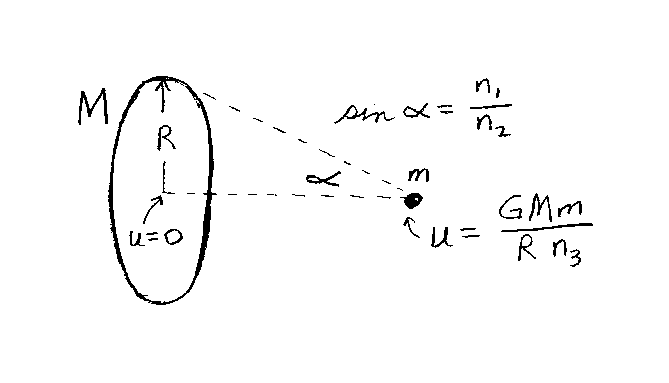Problem B10: Consider the two objects below. One is very small (point object) with mass m, and the other is a very thin circular ring of radius R and mass M. The object of mass m is located on the axis of the ring as shown in the figure. The angle α is the angle between the axis and a line drawn from the object to the ring. Note: sin(α)=n1/n2. What is the gravitational potential energy U of the mass and ring in the figure if U=0 when the object is at the center of the ring? If U=GMm/(Rn3) in the figure, what is n3? All the integers are unitless,n1 = n2 = Input n3:

If you are currently in my class, you can record your grade by entering your name and student ID number (without the leading zeros) below and clicking on "record grade".
 First Name = Last Name = ID = Problem: